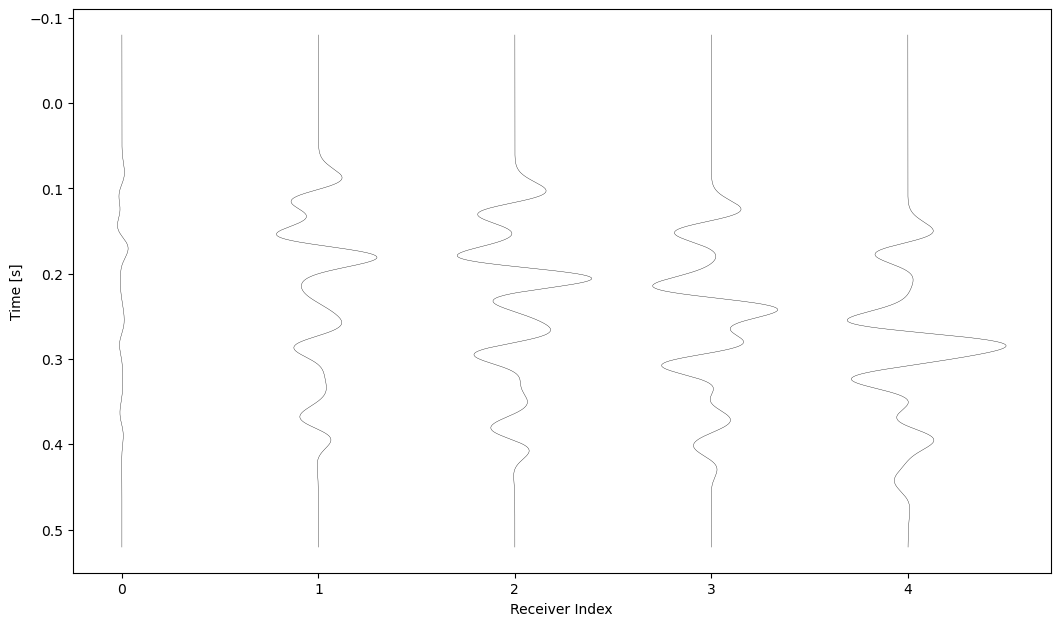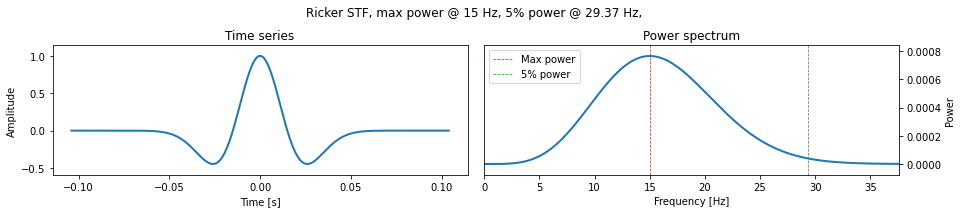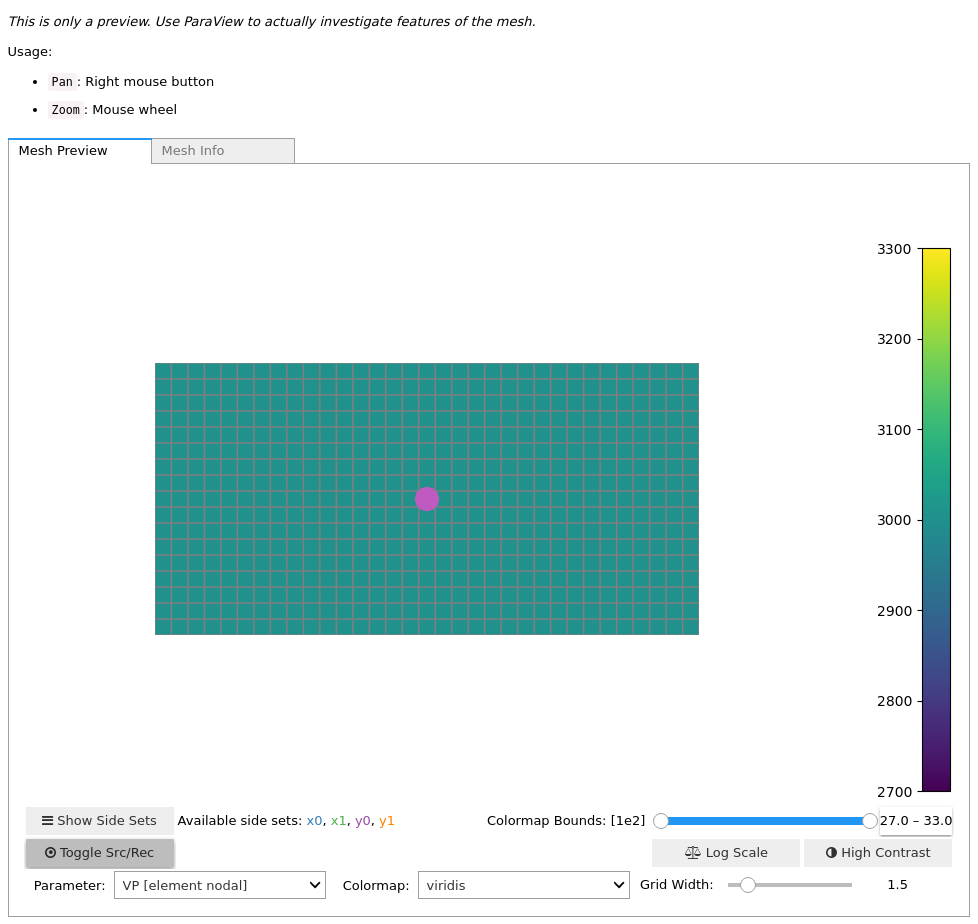Version:

This documentation is not for the latest stable Salvus version.This tutorial is presented as Python code running inside a Jupyter Notebook, the recommended way to use Salvus. To run it yourself you can copy/type each individual cell or directly download the full notebook, including all required files.Copy
%matplotlib inline
# This notebook will use this variable to determine which
# remote site to run on.
import os

SALVUS_FLOW_SITE_NAME = os.environ.get("SITE_NAME", "local")
PROJECT_DIR = "project"

# Lamb's problem

An accurate solution to the wave equation is a requirement for a wide variety of seismological research. In this tutorial, we will validate the accuracy of Salvus by comparing numerically calculated seismograms to semi-analytical solutions of Lamb's Problem in 2-D. In addition to giving us confidence in the synthetic data we will use in future tutorials, it also gives us a chance to gently learn some of the key features of the SalvusProject API.
Lamb's problem is concerned with the behavior of the elastic wave equation in the presence of a half-space bounded by a free-surface condition. In our solution we expect both direct arrivals and those reflected from the free-surface, along with a contribution from the 2-D Rayleigh wave. To validate the solutions generated with Salvus, we will compare our results with semi-analytical ones computed using EX2DDIR. We'll consider a half-space bounded at $y=2000$, and excite waves using a Ricker source with a center frequency of 15 Hz. This setup keeps compute times very low, while also allowing for a fair amount of wavelengths to propagate within our domain. To get started, let's first import all the Python tools we'll need.
import pathlib
import numpy as np
import salvus.namespace as sn

## Initializing a project

Before we initialize our project, we'll first need to initialize the spatial domain to which our project corresponds. In this case we'll be using a simple 2-D box domain. Don't worry, we'll make things a bit more exciting in future tutorials. The box domain can easily be constructed from a set of two dimensional extents as shown in the cell below.
d = sn.domain.dim2.BoxDomain(x0=0.0, x1=2000.0, y0=0.0, y1=1000.0)
For a given project the domain we specify is immutable; its extents and characteristics are used to infer other information regarding our meshes, simulations, and data. With the simple domain definition given above, we're now ready to initialize our project. To do this we can use the Project.from_domain() constructor as show below. This function takes a path (which must not yet exist), and a domain object such as the one we just constructed.
# Uncomment the following line to delete a
# potentially existing project for a fresh start
# !rm -rf proj
if pathlib.Path(PROJECT_DIR).exists():
print("Opening existing project.")
p = sn.Project(path=PROJECT_DIR)
else:
print("Creating new project.")
p = sn.Project.from_domain(path=PROJECT_DIR, domain=d)
Creating new project.

If the cell executed without any problems, you should now see a folder in your current directory with the name proj. This is where all the relevant information relating to your project will be stored. Just so we can get a hang of the basic structure of a project, let's open up the folder in our file browser. Most operating systems will understand the command below, and will open the project folder in another window. Just uncomment the line for your operating system.
# On Mac OSX
# !open proj

# On Linux:
# !xdg-open proj

## Adding observed data

With our domain initialized and our project created, we can now go right ahead and start preparing some scientific data. The first thing we'll do with the project is add observed data to it. In this case our observed data corresponds to a semi-analytic solution to Lamb's problem, as described in the introduction. These data are stored in an HDF5 file named reference_data.h5 in the current directory.
Some data formats, such as ASDF or SEGY describe their data with associated headers. We'll see how to add these types of data in a later tutorial, but in this case we are just reading in raw waveform traces with little to no meta information. Because of this we'll need to assist Salvus a little and tell the project to what events this raw data refers to. This information is passed in the form of an EventCollection object which, at is most basic, is a data structure which relates lists of source definitions to lists of receiver definitions. These definitions can be in the form of pressure injections, force vectors, or GCMT moment tensors for sources, as well as pressure, velocity, or strain (etc.) sensors for receivers. In the coordinate system of the reference dataset which we'll add, we've placed a single vector source at the location $(x=1000, y=500)$. This source can be defined with the help of the simple_config helper as in the cell below.
srcs = sn.simple_config.source.cartesian.VectorPoint2D(
x=1000.0, y=500.0, fx=0.0, fy=-1.0
)
The data from this source was received at an array of 5 receivers at locations $(x=\{1010, 1110, 1210, 1310, 1410\}, y=800)$. For these and other simple arrays of receivers, the simple_config helper allows us to define the set in one go.
recs = sn.simple_config.receiver.cartesian.collections.ArrayPoint2D(
y=800.0, x=np.linspace(1010.0, 1410.0, 5), fields=["displacement"]
)
With our sources and receivers now defined, we can add the combination of them both to our project as an EventCollection object.
p += sn.EventCollection.from_sources(sources=[srcs], receivers=recs)
Note here the syntax we used. An EventCollection, along with several other relevant objects, can be added to a project by simply using the += operator. Once the object is succesfully added to the project it is then "serialized", or saved, within the project directory structure. The power and usefulness of this concept will become apparent in a later tutorial -- for now all you need to know is that the event collection is now officially a part of our project!
Now that we've defined a full "event", we can go ahead and add our "observed" data. We do this by explicitly associating the event with the appropriate data file. Since the event does not have a natural name, as it would in the case of an event gathered from the GCMT catalogue for example, the project has named it for us internally. Events are given numerical names of the form "event_xxxx", which correspond to the order in which they were added. Below we add the reference data to our project with the tag "reference", and associate it with the event we just created, or "event_0000".
p.waveforms.add_external(
data_name="reference",
event="event_0000",
data_filename="./reference_data.h5",
)
Now that the data is added, we can do a quick visualization of its contents. For 2-D box domains we can choose to plot individual events as either a shotgather, or a collection or wiggles, or both! Try experimenting with the list passed to .plot() below to see how the different options look.
p.waveforms.get(data_name="EXTERNAL_DATA:reference", events=["event_0000"])[
0
].plot(component="X", receiver_field="displacement")All right, that's enough setup for now. Let's get going with some simulations of our own.

## Running a simulation

#### Defining a model

The analytical solution was computed in an unbounded homogeneous isotropic elastic medium with material parameters specified in SI units as $(\rho = 2200, v_p = 3000, v_s = 1847.5)$. If you recall from the presentation this morning, a complete model definition in Salvus is made up of a combination of a background model, and a (possibly empty) collection of volumetric models. As the analytic solution was computed in a homogeneous medium, we don't need to concern ourselves with (2- or 3-D) volumetric models for now. So, the next step is to define our background model using the salvus model interface. Since no volumetric models are required, we only need the background model to complete our final full model configuration.
bm = sn.model.background.homogeneous.IsotropicElastic(
rho=2200.0, vp=3000.0, vs=1847.5
)
mc = sn.ModelConfiguration(background_model=bm, volume_models=None)

#### Defining source parameters

Note that up until now we have not specified any information regarding the frequency content of the data we are planning on simulating, and in fact all the parameters we've specified have been frequency independent. This is deliberate, as it is often the case that information on material parameters are provided independent of frequency. The next step is to add a time-frequency axis to our project, which enters in the form of an EventConfiguration. Here, at a bare minimum, we need to specify what type of source wavelet we would like to model, as well provide some basic information about the temporal extent of our upcoming simulations. The reference data were computed with using a Ricker wavelet with a center frequency of $15 Hz$ and, looking at the traces plotted above, we can see that the data runs for a bit more than 0.5 seconds. These parameters are now used to define our EventConfiguration object.
ec = sn.EventConfiguration(
wavelet=sn.simple_config.stf.Ricker(center_frequency=15.0),
waveform_simulation_configuration=sn.WaveformSimulationConfiguration(
end_time_in_seconds=0.6
),
)
To get a better sense of what our wavelet looks like in both the time and frequency domain, we can easily plot its characteristics in the cell below.
ec.wavelet.plot()We quickly see that, while the center frequency of the wavelet was specified to be $15Hz$, there is actually a fair bit of energy that exists at frequencies higher than this. Its important to design our simulations so that they properly resolve all the frequencies we are interested in.

#### Defining simulation parameters

The final step in defining a simulation is pulling together all the above into a single reproducible SimulationConfiguration. A SimulationConfiguration is a unique identifier that brings together the model, the source wavelet parameterization, and a proxy of the resolution of the simulation together. If you recall from the theoretical presentations earlier today, we are often satisfied with a simulation mesh comprised of 1 4th order spectral-element per simulated wavelength. The question then remains: given a broadband source wavelet, which frequency do we want to mesh for? The wavelet plot above gives us a clue: the vast majority of the energy in the current wavelet is contained at frequencies below $30Hz$. For our first attempt at matching the analytic solution then, we'll require that our mesh be generated using one element per wavelength at a frequency of $30Hz$. As you are probably becoming familiar with by now, we can add the relevant SimulationConfiguration to our project as below.
p += sn.SimulationConfiguration(
name="simulation_1",
max_frequency_in_hertz=30.0,
elements_per_wavelength=1.0,
model_configuration=mc,
event_configuration=ec,
)
event = sn.EventCollection.from_sources(sources=[srcs], receivers=None)
w = p.simulations.get_input_files("simulation_1", events=event)
[2021-05-27 17:26:15,608] INFO: Creating mesh. Hang on.


#### Visualizing the configuration

So far, regarding our simulation, we have:
• Defined a homogeneous material model
• Defined a Ricker wavelet source
• Set a resolution criterion
In fact, this is all we need to do! Before we actually run the simulation though, it can be helpful to get a visual overview of what is about to happen. Salvus project provides a small convenience function to visualize a SimulationConfiguration directly in the notebook, as below. This function takes a list of events as well, for the purpose of overplotting sources and receivers on the resultant domain. Let's have a look.
w<salvus.flow.simple_config.simulation.Waveform object at 0x7f2747a3e450>
p.viz.nb.simulation_setup(
simulation_configuration="simulation_1", events=["event_0000"]
)